# What is ML Tooling

ML Tooling is a toolbox developed to help put machine learning models into production

It also has a number of quality-of-life functions to avoid repeated code across projects

• Datasets
• Plotting
• Pandas-friendly transformers
• Logging
• Saving estimators
• Hyperparameter Search

# Installation

``````pip install ml_tooling
``````

## Or

``````conda install ml_tooling
``````

# Ml-tooling ❤️ scikit-learn

ML Tooling is built on top of scikit-learn

This means that ML-Tooling is compatible with most Scikit-Learn workflows

We can use any estimator from Scikit-learn when creating our models

``````from ml_tooling import Model
from sklearn.ensemble import RandomForestClassifier

model = Model(RandomForestClassifier())
``````

We can directly implement any Scikit-learn pipelines/transformers we want

``````from ml_tooling import Model
from sklearn.pipeline import Pipeline
from sklearn.preprocessing import StandardScaler
from sklearn.ensemble import RandomForestClassifier

>>> pipe = Pipeline([
...    ("scaler", StandardScaler),
...    ("classifier", RandomForestClassifier())
...])

>>> model = Model(pipe)
<Model: RandomForestClassifier>
``````

(though to gain the full benefits, we should use ML-Tooling’s transformers)

# Datasets

To use ML Tooling, we need the `Model` and we need a `Dataset`

The `Dataset` represents our access to data and tells ML Tooling how to load data for training and prediction

A `Dataset` must implement two method

• `load_training_data`, which is expected to return a feature matrix and a target (X and y)

• `load_prediction_data`, which is expected to return a feature matrix and often accepts an ID of some kind to load data for that ID

As a general rule, we want everything in ML Tooling to be Pandas DataFrames

## Implementing a Dataset

``````from  ml_tooling.data import Dataset
from sklearn.datasets import load_iris

class IrisDataset(Dataset):
def load_training_data(self):
"""Implement how to load data when predicting"""
# Load iris as dataframes
iris_data = load_iris(as_frame=True)
return iris_data.data, iris_data.target

def load_prediction_data(self, idx):
"""Implement how to load data when predicting"""
iris_data = load_iris(as_frame=True)
return iris_data.data.iloc[[idx]]
``````

## We can now access the data

``````>>> iris_data = IrisDataset()
>>> iris_data.x
sepal length (cm)  sepal width (cm)  petal length (cm)  petal width (cm)
0                  5.1               3.5                1.4               0.2
1                  4.9               3.0                1.4               0.2
2                  4.7               3.2                1.3               0.2
3                  4.6               3.1                1.5               0.2
4                  5.0               3.6                1.4               0.2
..                 ...               ...                ...               ...
145                6.7               3.0                5.2               2.3
146                6.3               2.5                5.0               1.9
147                6.5               3.0                5.2               2.0
148                6.2               3.4                5.4               2.3
149                5.9               3.0                5.1               1.8

[150 rows x 4 columns]

``````

The first time `x` or `y` is accessed, ML Tooling calls `load_training_data` and then caches the result.

`load_training_data` is only ever called once.

## Convenience Dataset

The two most common usecases is loading data from a file or loading from a database

ML Tooling ships with two `Dataset` implementations to help with these usecases

• FileDataset
• SQLDataset

# FileDataset

Let’s dump our data to a parquet file, because csv’s are bad 😄

The parquet file will contain our data and target

``````import pandas as pd

# Make sure pyarrow is installed -> pip install pyarrow
pd.concat([load_iris(as_frame=True).data,
load_iris(as_frame=True).target],
axis=1).to_parquet("iris.parquet")
``````

Our FileDataset will accept a filepath which we can use in our loading logic

``````class FileIrisData(FileDataset):
def load_training_data(self):
data = self.read_file()
return data.drop(columns="target"), data.target

def load_prediction_data(self, idx):
data = self.read_file()
return data.drop(columns="target").iloc[[idx]]

``````

We can now point our FileDataset at the file we want to load

``````>>> file_data = FileIrisData("iris.parquet")
>>> file_data.x.head()
sepal_length  sepal_width  petal_length  petal_width
0           5.1          3.5           1.4          0.2
1           4.9          3.0           1.4          0.2
2           4.7          3.2           1.3          0.2
3           4.6          3.1           1.5          0.2
4           5.0          3.6           1.4          0.2

``````

# SQLDataset

SQLDataset is used when connecting to a database to load data. Let’s create a local sqlite database

``````import pandas as pd
import sqlalchemy as sa

engine = sa.create_engine("sqlite:///iris.db")

(pd.concat([load_iris(as_frame=True).data,
load_iris(as_frame=True).target],
axis=1)
# Make some more friendly column names
.rename(columns=lambda x: x.rsplit(" ", 1)
.replace(" ", "_")
.to_sql("iris", engine)
)
``````

## Creating a SQLDataset

``````from ml_tooling.data import SQLDataset

class SQLIrisData(SQLDataset):
table = sa.Table(
"iris",
sa.MetaData(),
sa.Column("index", sa.Integer, primary_key=True),
sa.Column("sepal_length", sa.Float),
sa.Column("sepal_width", sa.Float),
sa.Column("petal_length", sa.Float),
sa.Column("petal_width", sa.Float),
sa.Column("target", sa.Integer)
)
def load_training_data(self, conn):
select_statement = sa.select([self.table])
data = pd.read_sql(select_statement,
conn,
index_col="index")
return data.drop(columns="target"), data.target

def load_prediction_data(self, idx, conn):
select_statement = (
sa.select([self.table.c.sepal_length,
self.table.c.sepal_width,
self.table.c.petal_length,
self.table.c.petal_width])
.where(self.table.c.index == idx)
)
return pd.read_sql(select_statement, conn)
``````

To use it, pass a conn string and what schema to use

(sqlite doesn’t have schemas, so we set it to None)

``````>>> sql_data = SQLIrisData(conn="sqlite:///iris.db", schema=None)
>>> sql_data.x.head()
sepal_length  sepal_width  petal_length  petal_width
index
0               5.1          3.5           1.4          0.2
1               4.9          3.0           1.4          0.2
2               4.7          3.2           1.3          0.2
3               4.6          3.1           1.5          0.2
4               5.0          3.6           1.4          0.2
``````

## Copying data

One thing we can do with datasets, is to copy them between representations

(Make sure we delete our `iris.parquet` file)

``````import os
>>> os.remove('iris.parquet')
``````

We can copy data from a SQLDataset to a FileDataset

``````new_file_data = sql_data.copy_to(file_data)
[13:24:55] - Copying data...
[13:24:55] - Dumping data from iris
[13:24:55] - Data dumped...
>>> new_file_data.x.head()
index  sepal_length  sepal_width  petal_length  petal_width
0      0           5.1          3.5           1.4          0.2
1      1           4.9          3.0           1.4          0.2
2      2           4.7          3.2           1.3          0.2
3      3           4.6          3.1           1.5          0.2
4      4           5.0          3.6           1.4          0.2
``````

Or from one SQLDataset to another if we need to move data between databases

``````>>> other_sql_data = SQLIrisData("sqlite:///other_iris.db",
schema=None)
>>> sql_data.copy_to(other_sql_data)
[14:55:55] - Copying data...
[14:55:55] - Dumping data from iris
[14:55:55] - Data dumped...
[14:55:55] - Inserting data into iris
``````

# Plotting

ML Tooling comes with a number of plotting facilities to understand our data and models better

## Dataset plotting

We can plot some basic information about our dataset that we implemented previously

### Target correlation

``````>>> iris_data.plot.target_correlation()
``````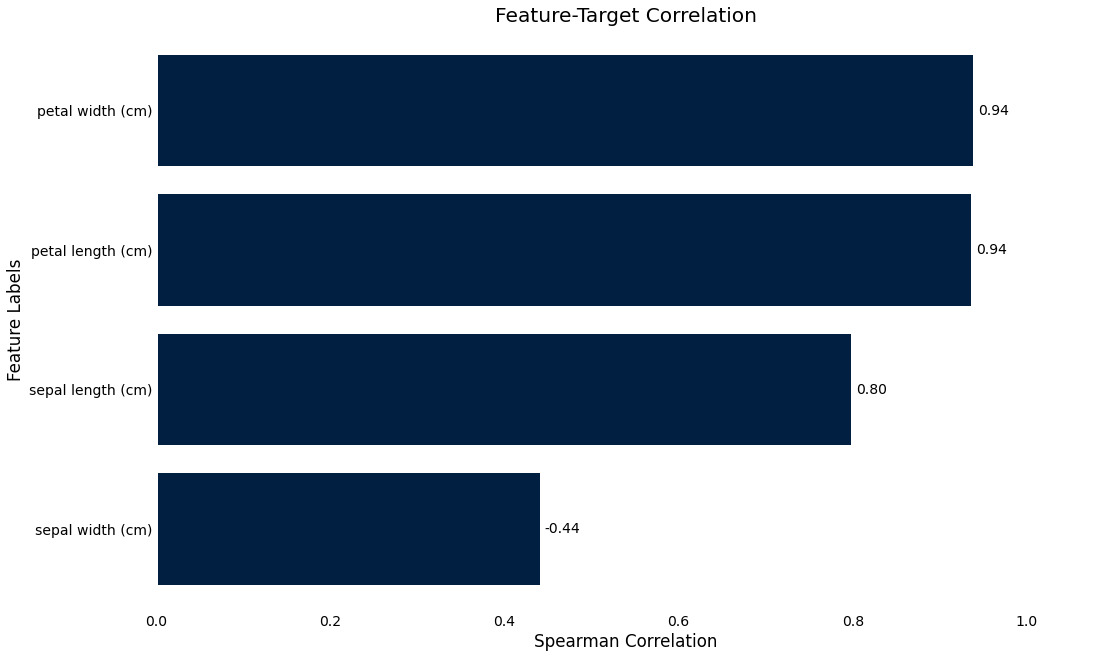### Missing data

This will show an overview of what data is missing in the dataset

``````>>> iris_data.plot.missing_data()
``````

## Result plots

To understand our model better, first we need to train a model. This will give us a `Result` object, which gives us access to the plotting functionality.

``````from ml_tooling import Model
from sklearn.ensemble import RandomForestClassifier

>>> model = Model(RandomForestClassifier())
>>> result = model.score_estimator(iris_data)
[15:15:35] - Scoring estimator...
``````

Note that all plots shown here have a `plot_*` counterpart to use if you want more finegrained control

When we train a model, we get back a `Result` object

``````>>> result
<Result RandomForestClassifier: {'accuracy': 0.89}>
``````

The `Result` represents a scoring of the estimator and contains information about the metrics used for scoring and their results, as well as a number of convenience plotting functions

We can also do cross-validated scoring by passing a `cv` parameter

``````>>> result = model.score_estimator(iris_data, cv=10)
[15:23:17] - Scoring estimator...
[15:23:17] - Cross-validating...
>>> result
<Result RandomForestClassifier: {'accuracy': 0.96}>
``````

We can now inspect the results by using the `Results.plot` accessor

## Confusion Matrix

We can plot a confusion matrix, with the option of normalizing

``````>>> result.plot.confusion_matrix(normalized=False)
``````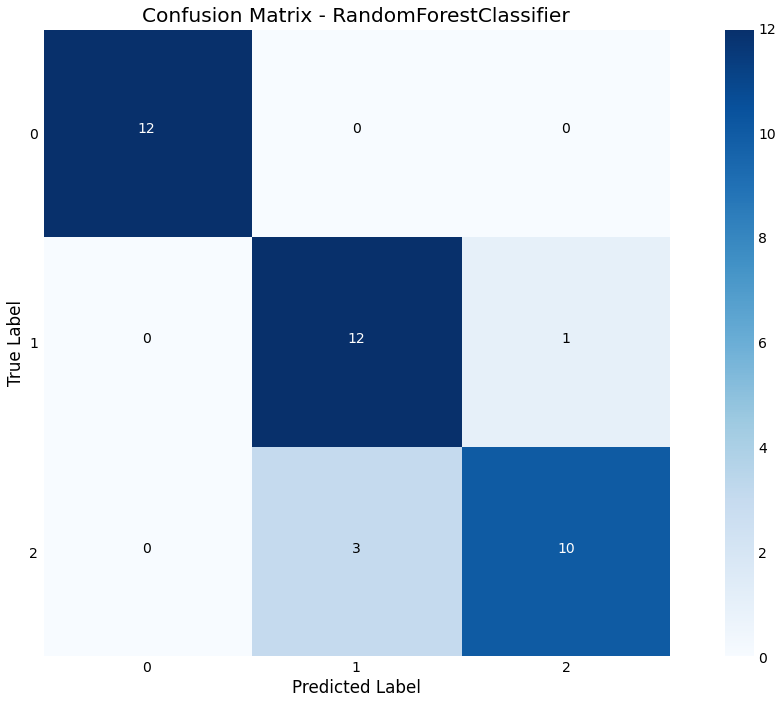## Feature Importance

We can plot feature importance based on either the model coefficients or the `RandomForest` feature_importance

``````>>> result.plot.feature_importance()
``````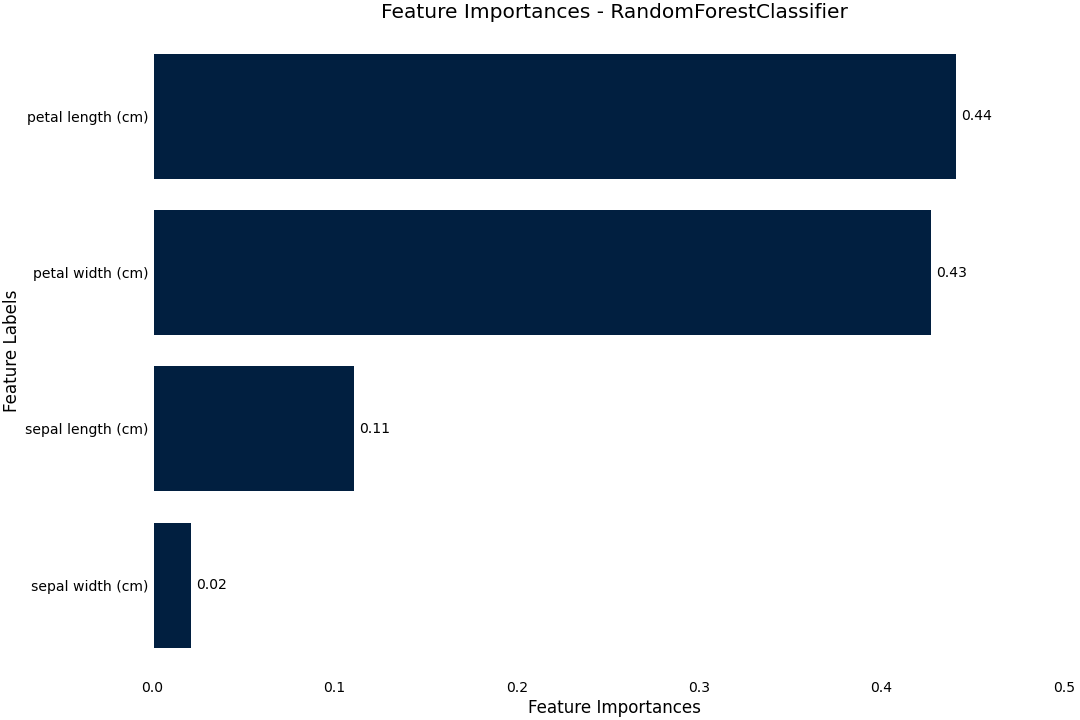## Permutation importance

We can also use the more precise, but more costly `permutation_importance` where we permute each column and compare the resultant score to the baseline

``````>>> result.plot.permutation_importance()
``````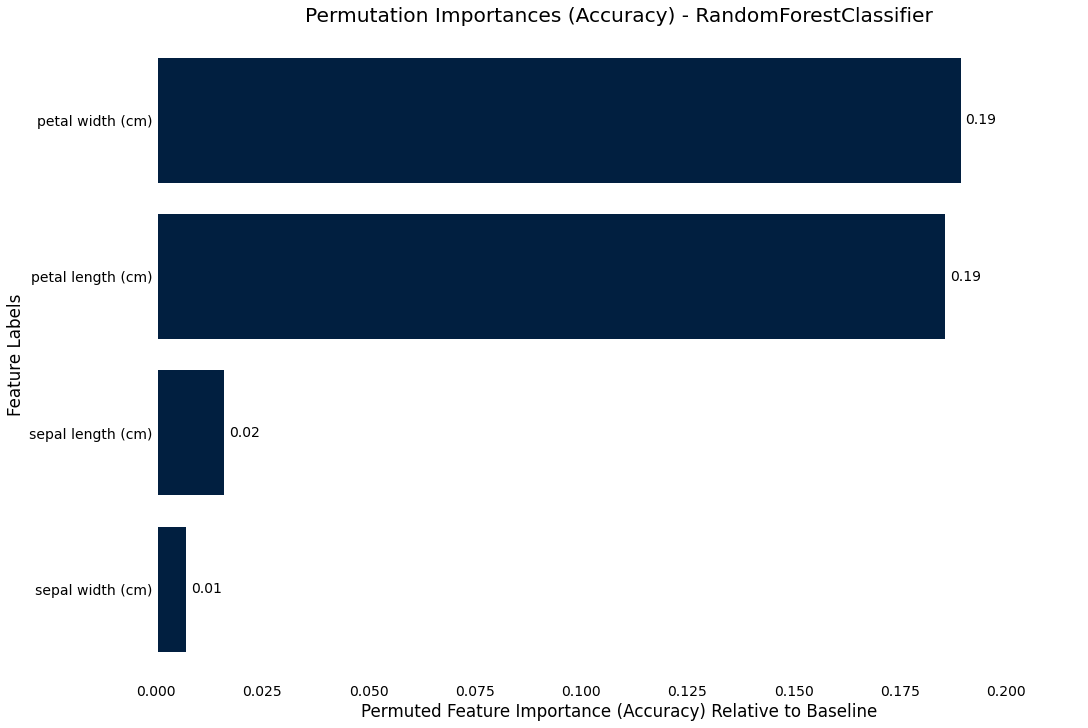## Precision-Recall curve

The precision-recall curve shows us how we are trading off precision and recall in the estimator across different thresholds

``````>>> result.plot.precision_recall()
``````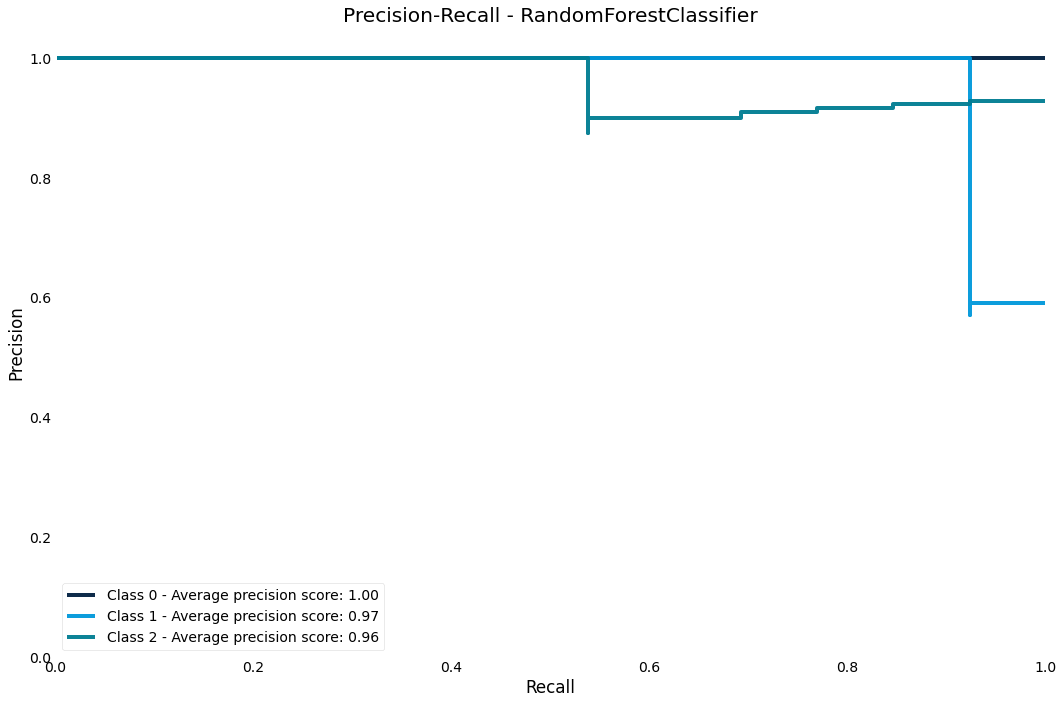## ROC AUC curve

The ROC curve is another classic performance plot for classification - we should generally always check the ROC of a classifier

``````>>> result.plot.roc_auc()
``````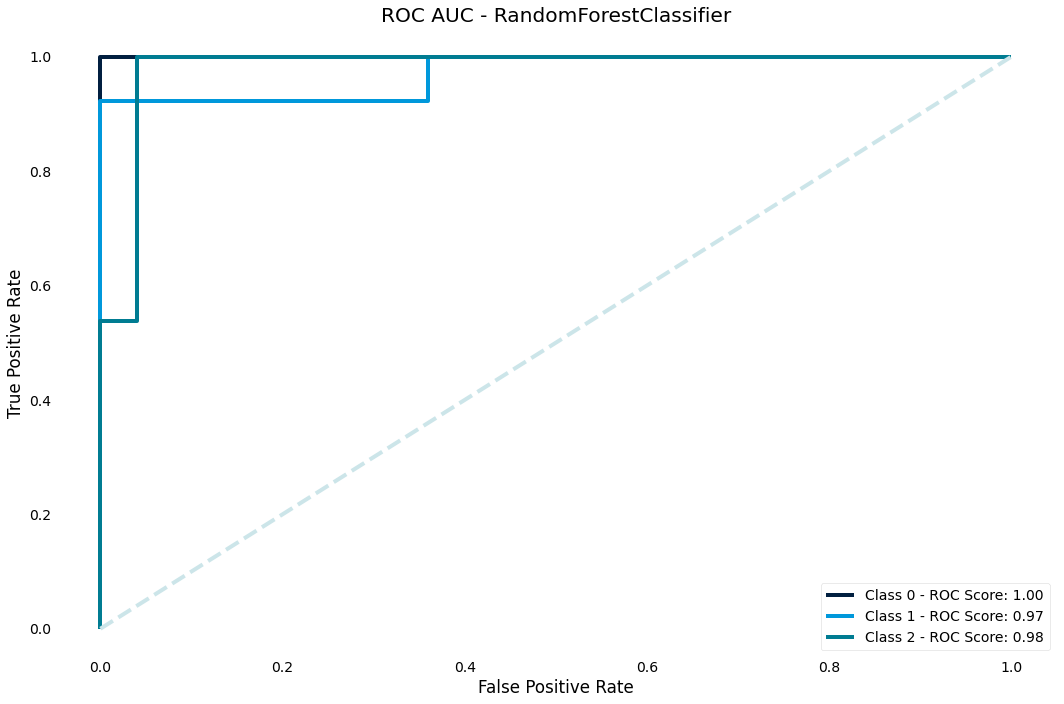## Lift score

The lift score will show us how much better our model is than random guessing

``````>>> result.plot.lift_curve()
``````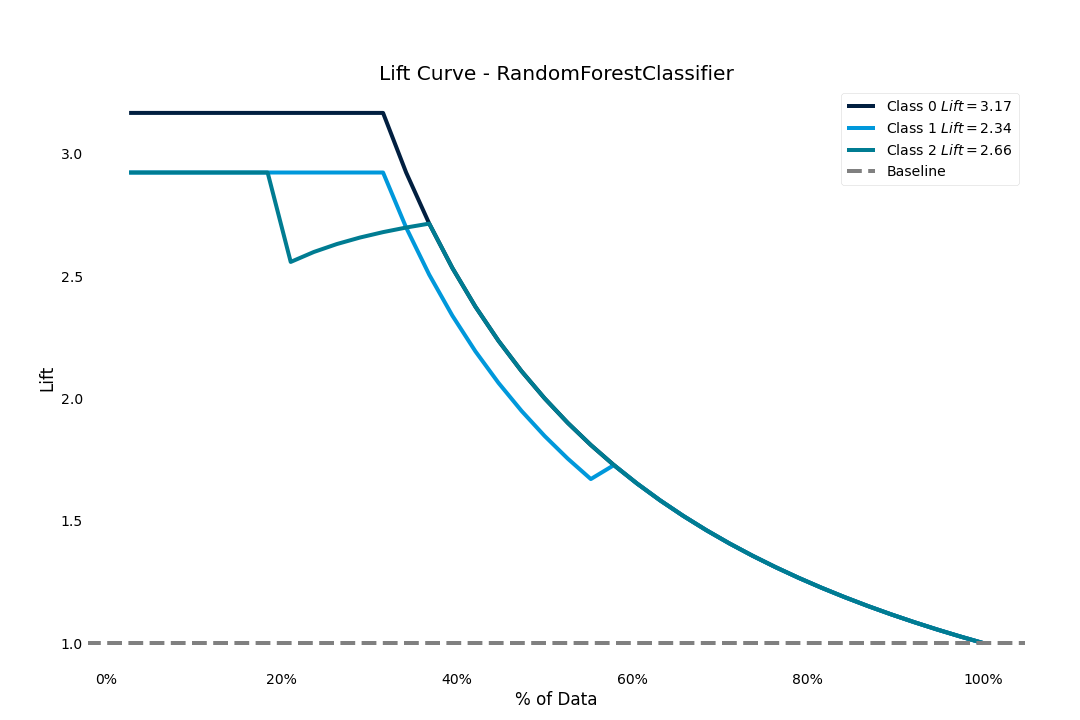## Learning curve

Another important chart is the learning curve - we use it to diagnose whether our model is under or overfitting and if we need to increase or decrease complexity

``````>>> result.plot.learning_curve()
``````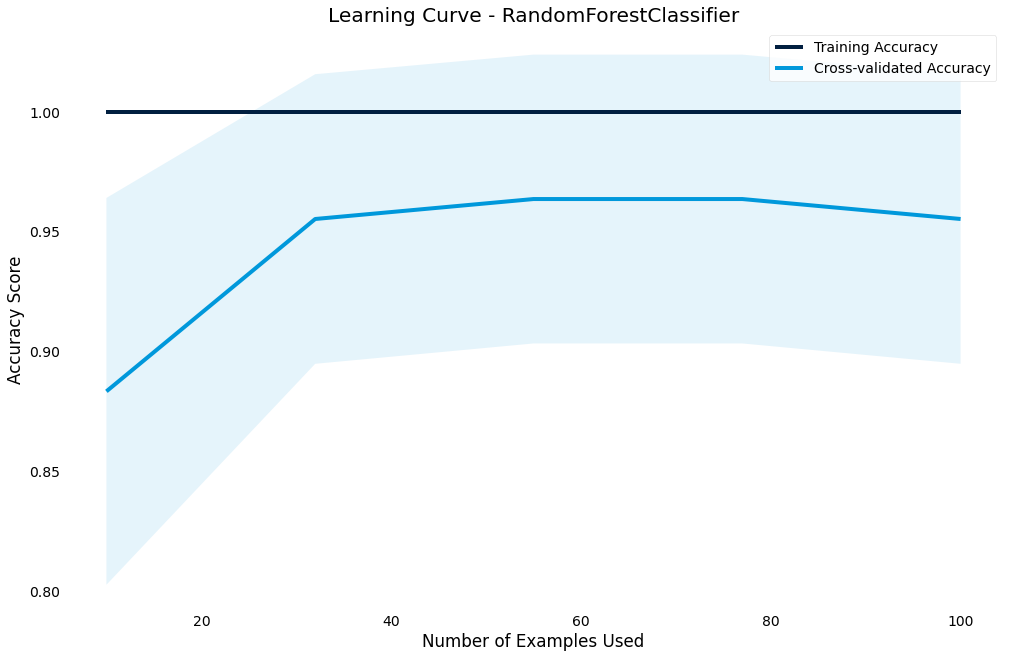## Validation curve

The validation curve lets you plot the performance of the model against a hyperparameter.

It shows effect of the hyperparameter on the model and gives an intuition for how the model responds to that parameter

``````>>> result.plot.validation_curve("max_depth",
param_range=[1, 5, 10, 20, 30, 40, 60, 80, 100])
``````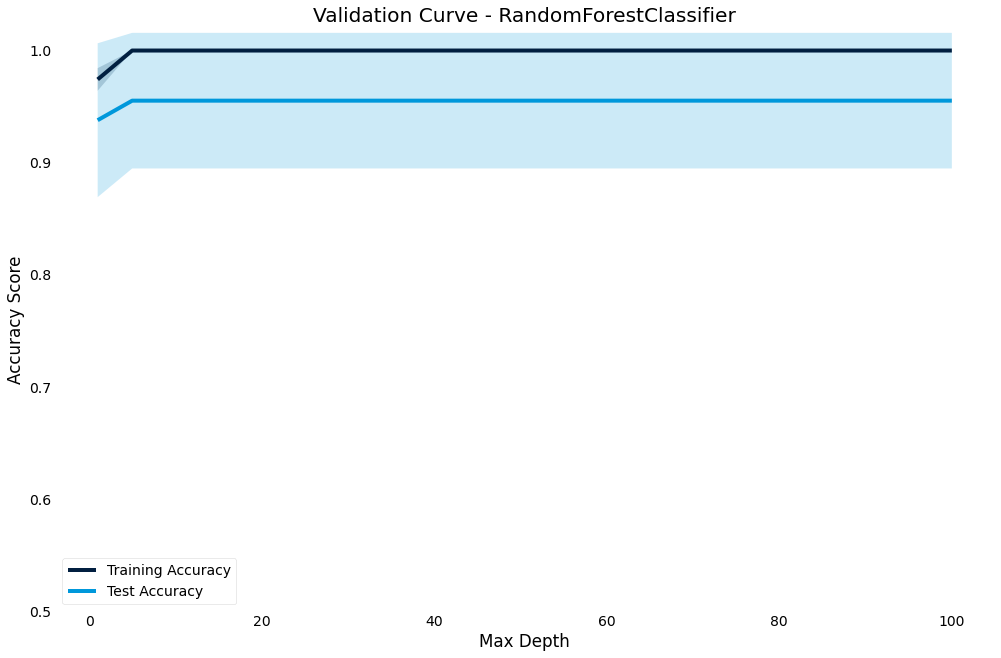# Regression

If we have a regression problem, the plots available will be different, although some will be available for both types

``````from ml_tooling.data import load_demo_dataset
from ml_tooling import Model
from sklearn.ensemble import RandomForestRegressor

>>> dataset = load_demo_dataset("boston")
>>> model = Model(RandomForestRegressor())
>>> result = model.score_estimator(dataset)
``````

## Residual plot

To check for goodness-of-fit, we can check the residual fit to verify that the residuals seem randomly distributed

``````>>> result.plot.residuals()
``````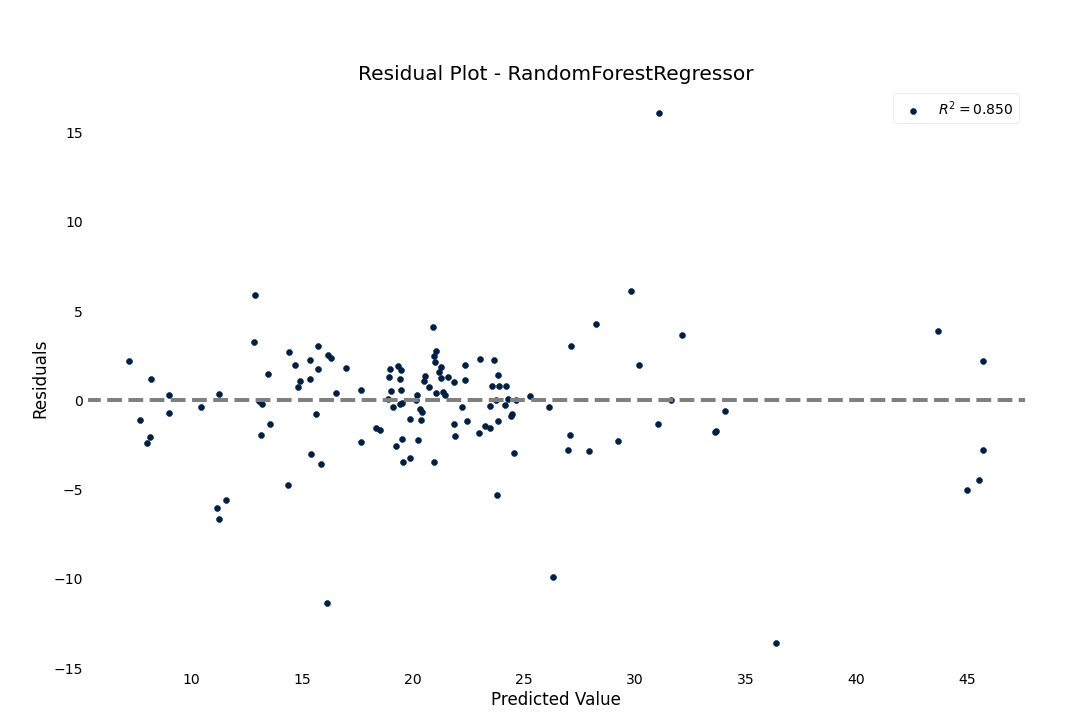## Prediction Error

We can also see how good the regression model is by plotting the predictions against the target

``````>>> result.plot.prediction_error()
``````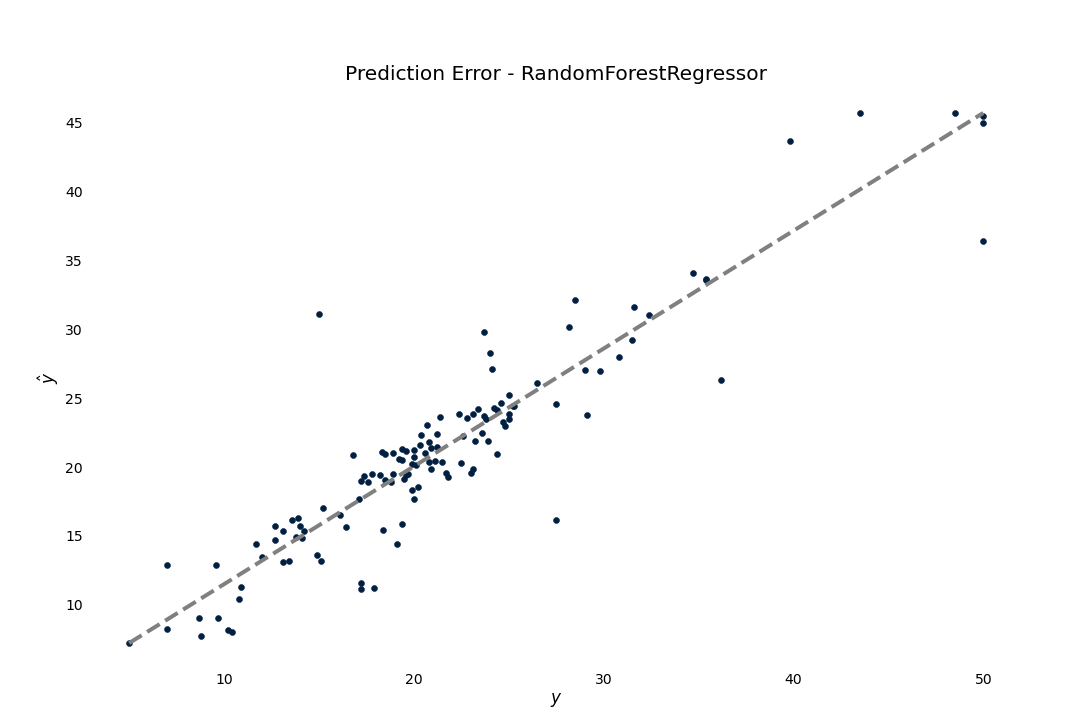# Transformers

• Everything in ML Tooling is based around pandas `DataFrames`
• This lets us pass metadata such as column names to our functions and methods.
• Thus, we need to implement DataFrame-friendly transformers in ML-Tooling

## The importance of Pipelines

• The `Pipeline` is the foundation of building robust preprocessing in scikit-learn.
• It lets us specify our entire data pipeline as a single object
• Additionally, scikit-learn makes sure to only learn attributes about your data when training, so that no accidental data leakage occurs.

## An overview of transformers

• All ML Tooling transformers live in `ml_tooling.transformers`
• It’s simple to implement your own, and is considered part of the scikit-learn toolkit
• The documentation has plenty on the available transformers

## A typical pipeline

We want to set up a Pipeline describing what features we want to use, how to preprocess them and join them together

### Define our features

``````from ml_tooling.transformers import (
Pipeline,
DFFeatureUnion,
Select,
FillNA,
ToCategorical,
DFStandardScaler)

age = Pipeline([
("select", Select("age")),
("fillna", FillNA(strategy="mean", indicate_nan=True))
])

house_type = Pipeline([
("select", Select("house_type")),
("fillna", FillNA("missing", indicate_nan=True)),
("categorical", ToCategorical())
])

numerical = Pipeline([
("select", Select(["customer_since_days",
"car_probability",
"profitability"])),
("scale", DFStandardScaler())
])
``````

### Combine our features

``````feature_pipeline = DFFeatureUnion([
("age", age),
("housetype", house_type),
("numerical", numerical)
])

>>> feature_pipeline.fit_transform(train_x)
``````

### Define our Model

``````>>> model = Model(RandomForestClassifier(),
feature_pipeline=feature_pipeline)
``````

## Exercise

• Setup a pipeline for the FileDataset we made
• Train a model on the data
• Explore the plots

# Logging

## Log what our models do

• We can log the output of our models, so that we can keep track of what we have done previously
• ML Tooling can automatically keep track of the models we train if we turn on logging
``````>>> model = Model(RandomForestClassifier())
>>> with model.log("textclassifier"):
model.score_estimator(dataset)
``````

This will create a folder named “runs” with a yaml file inside

``````created_time: 2020-08-07 12:23:21.470576
estimator:
// The entire pipeline definition...
- classname: RandomForestClassifier
module: sklearn.ensemble._forest
name: estimator
params:
// All the parameters...
estimator_path: null
git_hash: ''
metrics:
accuracy: 0.8333333333333334
model_name: SchoolPlacement_RandomForestClassifier
versions:
ml_tooling: 0.11.0
pandas: 1.1.0
sklearn: 0.23.1
``````

We can reload the defined model from the saved yaml if we decide we want to retry a given model

``````>>> model = Model.from_yaml("./runs/placement/SchoolPlacement_RandomForestClassifier_130257_0.yaml")
``````

# Saving models

## Storing your estimators

We can save our models as pickle files to reuse later or to put in production

## Storage

We provide two Storage classes that let you store the model

• FileStorage
• ArtifactoryStorage

Create an instance of `Storage` and pass it to `model.save_estimator`

``````>>> storage = FileStorage("./my_models")
>>> model.save_estimator(storage)
[13:13:55] - Saved estimator to estimators/RandomForestClassifier_2020_08_07_13_13_55_137029.pkl
``````

We can also log the saved estimator - this saves the estimator filepath to the log as well

``````>>> with model.log("classifier"):
...     model.save_estimator(fs)
``````

### ArtifactoryStorage

`ArtifactoryStorage` works similarly to `FileStorage` expect we need to instantiate it with the url, repo and authorization

``````storage = ArtifactoryStorage('http://artifactory.com',
repo='classifier_project',
api_key="MYAPIKEYHERE")
``````

It can then be used just like FileStorage, but it will save and load estimators in Artifactory instead.

(Must have installed with the artifactory optional dependency - ml_tooling[artifactory])

## Storing a production model

When you are ready to productionize your model we must

• Train the final estimator
• Save production estimator
``````# This will train a final model on all of X - no train-test split
>>> model.train_estimator()
>>> model.save_estimator(prod=True) # This will only work in a production package setting!
``````
• A production estimator expects you to be working in a python package
• It looks for a setup.py/pyproject.toml file to establish the root of your project and puts the pickle file in the src folder
• If these don't exist then it will fail
• Make sure to include the pkl file in your package data before publishing your package!

## Load production model

If you have installed an ML Tooling based package that has saved a production model correctly, we can load that model

``````model = Model.load_production_estimator("name_of_model_package")
``````

This will load the production estimator from the python package

# Searching

ML Tooling implements three hyperparameter search options

• Gridsearch
• Randomsearch
• Bayesiansearh

## Gridsearch

Classic gridsearch

``````>>> param_grid = {"estimator__max_depth": [1, 2, 4, 8, 16, 32, 64]}
>>> best_model, results = model.gridsearch(dataset,
param_grid=param_grid)
``````

We use gridsearch to explore the hyperparameter space systematically

• Focus on one hyperparameter at a time
• Start with a wide range and narrow it down

## Randomsearch

• Randomsearch is used to cover a larger hyperparameter space with the same “budget”
• This is often a good first pass if we have no prior knowledge of where to search

To use, we specify distributions to sample from using Space objects from skopt

``````from ml_tooling.search import Integer

>>> param_distributions={"estimator__max_depth": Integer(1, 200)}
>>> best_estimator, results = model.randomsearch(
dataset,
param_distributions=param_distributions)
``````

By default, we run 10 trials, but can change that with the `n_iter` parameter

## Bayesiansearch

• Often more effective than Randomsearch, but can be more expensive since it cannot be parallelized
• Uses the results of the previous result to guide the choice of the next hyperparameter from the distributions
``````>>> param_distributions = {"estimator_max_depth": Integer(1, 200)}
>>> best_estimator, results = model.bayesiansearch(
dataset,
param_distributions=param_distributions)
``````

Like randomsearch, bayesiansearch runs 10 trials by default

## ResultGroups

For all the searches we get back a `ResultGroup`- a container for `Results`.

``````best_estimator, results = model.bayesiansearch(dataset,
param_distributions={"estimator__max_depth": Integer(1, 200)},
metrics=["accuracy", "roc_auc"],
n_iter=2)
>>> results
ResultGroup(results=[
<Result RandomForestClassifier: {'accuracy': 0.85, 'roc_auc': 0.93}>,
<Result RandomForestClassifier: {'accuracy': 0.84, 'roc_auc': 0.94}>])
``````

The ResultGroup sorts by the first metric passed, but we can change the sorting, either by changing the order passed or by calling sort

``````>>> result.sort(by="roc_auc")
ResultGroup(results=[
<Result RandomForestClassifier: {'accuracy': 0.84, 'roc_auc': 0.94}>,
<Result RandomForestClassifier: {'accuracy': 0.85, 'roc_auc': 0.93}>])
``````

Attribute access is delegated to the first result - otherwise we have to index into the ResultGroup

``````>>> results.metrics # We get the first result's metrics
Metrics(metrics=[
Metric(name='accuracy', score=0.8444852941176471),
Metric(name='roc_auc', score=0.9356060606060606)])
>>> results.metrics
Metrics(metrics=[
Metric(name='accuracy', score=0.8511029411764707),
Metric(name='roc_auc', score=0.931060606060606)])
``````

Remember, we can log searches too - we get one log per model trained

``````param_distributions = {"estimator__max_depth": Integer(1, 200)}

with model.log("search"):
best_estimator, results = model.bayesiansearch(
dataset,
param_distributions=param_distributions,
metrics=["accuracy", "roc_auc"],
n_iter=2)
``````

## Final Assignment

Build the best model you can on the data

• Try some different hyperparameter searches
• Log some results
• Save the best estimators

# Additional Resources

## Issues

If any issues arise, make sure to file an issue here